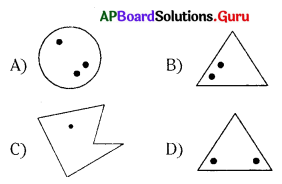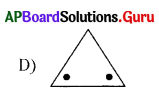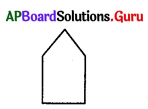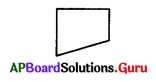Practice the AP 7th Class Maths Bits with Answers Chapter 15 Symmetry on a regular basis so that you can attempt exams with utmost confidence.

## AP State Syllabus 7th Class Maths Bits 15th Lesson Symmetry with Answers

Question 1.
Which of the following figure has axes of symmetry?Question 2.
Number of axes of symmetry for a circle.
A) 1
B) 2
C) 0
D) Infinite
D) InfiniteQuestion 3.
The angle of a rotational symmetry of a square
A) 60°
B) 45°
C) 90°
D) 30°
C) 90°

Question 4.
What is the angle of a rotational symmetry of a parallelogram?
A) 180°
B) 90°
C) 45°
D) 120°
A) 180°

Question 5.
Which of the following figure has symmetry?
A) Maths box
B) Cricket ball
C) A wheel
D) All the above
D) All the above

Question 6.
Which of the following has rotational symmetry?
A) Wheel
B) Square
C) Circle
D) All the above
D) All the above

Question 7.
Order of rotational symmetry of ‘X’ is …………….
A) 4
B) 2
C ) 3
D) 6
A) 4

Question 8.
Rotational angle of an equilateral triangle is …………….
A) 90°
B) 120°
C) 60°
D) 30°
B) 120°

Question 9.
Number of axes of symmetry of a letter ‘M’ is ………………
A) 0
B) 2
C) 1
D) 3
C) 1Question 10.
What are the minimum number of sides of a polygon?
A) 1
B) 2
C) 3
D) 4
C) 3

Question 11.
Which of the following triangle has 2 lines of symmetry?
A) Scalene
B) Right angled
C) Equilateral
D) Isosceles
D) Isosceles

Question 12.
Number of lines of symmetry for an equilateral triangle are?
A) 1
B) 2
C) 3
D) 4
C) 3

Question 13.
Number of lines of symmetry for a square are ……………..
A) 4
B) 3
C) 2
D) 5
A) 4

Question 14.
Which of the following statement is wrong?
A) Every square has an axes of symmetry
B) The letter ‘Z’ has no line of symmetry
C) The letter ‘E’ has no rotational symmetry
D) Every figure has an axes of symmetry
D) Every figure has an axes of symmetry

Question 15.
Order of rotational symmetry of ‘N’ is ……………….
A) 1
B) 2
C) 3
D) 4
B) 2

Question 16.
A square has ……………. lines of symmetry.
A) 1
B) 2
C) 3
D) 4
D) 4Question 17.
A circle has …………… lines of symmetry.
A) 0
B) 1
C) 4
D) inifinity
D) inifinity

Question 18.
Which of the following has a line symmetry?
A) P
B) F
C) M
D) Q
C) M

Question 19.
The angle of rotational symmetry of an equilateral triangle is
A) 60°
B) 120°
C) 180°
D) 360°
B) 120°

Question 20.
The order of rotational symmetry of an equilateral triangle is
A) 3
B) 2
C) 1
D) 4
A) 3

Question 21.
The order of rotational symmetry of a regular hexagon is
A) 6
B) 4
C) 3
D) 1
A) 6

Question 22.
Angle between successive axes of symmetry
A) $$\frac{90}{\mathrm{n}}$$
B) $$\frac{90}{2 \mathrm{n}}$$
C) $$\frac{360}{2 \mathrm{n}}$$
D) $$\frac{360}{\mathrm{n}}$$
C) $$\frac{360}{2 \mathrm{n}}$$

Question 23.
The line which divides a figure into two identical parts is called
A) axis of symmetry
B) point of symmetry
C) rotational symmetry
D) None
A) axis of symmetryQuestion 24.
Number of lines of symmetry for ‘H’
A) 2
B) 1
C) 3
D) 4
A) 2

Question 25.
Which letter has no line of symmetry?
A) A
B) B
C) C
D) F
D) F

Question 26.
Number of axes of symmetry of?
A) 1
B) 2
C) 3
D) 4
A) 1

Question 27.
Number of axes of symmetry of?
A) 1
B) 2
C) 3
D) 4
D) 4

Question 28.
Number of axes of symmetry of?
A) 1
B) 2
C) 3
D) 4
B) 2

Question 29.
Number of axes of symmetry of?
A) 2
B) 3
C) 4
D) 5Number of axes of symmetry of?